Updating search results...

# 11 Results

View
Selected filters:
• NC.M3.F-TF.5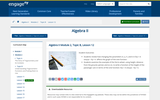Conditional Remix & Share Permitted
CC BY-NC-SA
Rating
0.0 stars
Overview:

Students review how changing the parameters A, ω, h, and k in f(x) = A sin(ω(x - h)) + k affects the graph of the sine function.
Students examine the example of the Ferris wheel, using height, distance from the ground, period, and so on, to write a function of the height of the passenger cars in terms of the sine function: f(x) = A sin(ω(x - h)) + k.

Subject:
Math 3
Material Type:
Lesson Plan
Author:
EngageNY
02/23/2020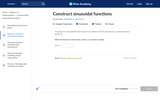Rating
0.0 stars
Overview:

In this Khan Academy activity, students will construct sinusoidal functions given information about it.

Subject:
Mathematics
Material Type:
Interactive
Provider:
09/06/2018
Remix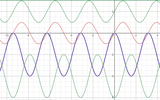Unrestricted Use
CC BY
Rating
0.0 stars
Overview:

This is an exploration activity for students to visually see the graph of trig functions and understand about sin and cos functions and understand the concept of amplitude.Section 2 of this activity allows students to explore the period and vertical shift in trig functions.

Subject:
Mathematics
Math 3
Pre-Calculus
Trigonometry
Material Type:
Activity/Lab
Author:
VIJAYALAKSHMI SANKARAN
05/28/2020Unrestricted Use
CC BY
Rating
0.0 stars
Overview:

This is an exploration activity for students to visually see the graph of trig functions and understand about sin and cos functions and understand the concept of amplitude.

Subject:
Mathematics
Math 3
Pre-Calculus
Trigonometry
Material Type:
Activity/Lab
Author:
VIJAYALAKSHMI SANKARAN
05/27/2020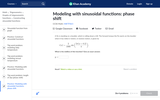Rating
0.0 stars
Overview:

In this Khan Academy activity, students will model word problems with sinusoidal functions that have a phase shift.

Subject:
Mathematics
Material Type:
Interactive
Provider:
09/06/2018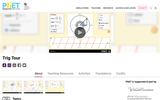Unrestricted Use
CC BY
Rating
0.0 stars
Overview:

Sample Learning Goals
Define trig functions for negative angles and angles greater than 90 degrees.
Translate between multiple representations of trig functions: as sides of a right triangle inscribed in a unit circle, graph of the function vs. angle, and numerical values of the function.
Deduce the sign (+, -, 0) of a trig function for any given angle without a calculator using the unit circle concept.
Estimate the value of trig functions for any given angle without a calculator using the unit circle concept.
Define exact trig functions for special angles using degrees or radians for angle measures.

Subject:
Science
Material Type:
Activity/Lab
Author:
PhET Interactive Simulations
10/05/2021Conditional Remix & Share Permitted
CC BY-NC
Rating
2.0 stars
Overview:

CK-12 Foundation's Trigonometry FlexBook is an introduction to trigonometry for the high school student. It includes chapters on graphs of trigonometric functions, trigonometric identities, inverse trigonometric functions, triangles and vectors, and the polar system.

Subject:
Mathematics
Material Type:
Textbook
Provider:
CK-12 Foundation
Provider Set:
CK-12 FlexBook
12/06/2018Conditional Remix & Share Permitted
CC BY-NC-SA
Rating
2.0 stars
Overview:

CK-12 Foundation's Trigonometry FlexBook is an introduction to trigonometry for the high school student. Topics include: Trigonometric Identities & Equations, Circular Functions, and Polar Equations & Complex Numbers.

Subject:
Mathematics
Material Type:
Textbook
Provider:
CK-12 Foundation
Provider Set:
CK-12 FlexBook
Author:
Fortgang, Art
Hayes, Andrea
Jordan, Lori
Landers, Mara
Meery, Brenda
Ottman, Larry
08/12/2010Conditional Remix & Share Permitted
CC BY-NC-SA
Rating
2.0 stars
Overview:

CK-12's Texas Instruments Trigonometry Student Edition Flexbook is a helpful companion to a trigonometry course, providing students with more ways to understand basic trigonometric concepts through supplementary exercises and explanations.

Subject:
Mathematics
Material Type:
Textbook
Provider:
CK-12 Foundation
Provider Set:
CK-12 FlexBook
Author:
Jordan, Lori
12/14/2010Conditional Remix & Share Permitted
CC BY-NC-SA
Rating
2.0 stars
Overview:

CK-12's Texas Instruments Trigonometry Teacher's Edition Flexbook is a helpful companion to a trigonometry course, providing students with more ways to understand basic trigonometric concepts through supplementary exercises and explanations.

Subject:
Mathematics
Material Type:
Textbook
Provider:
CK-12 Foundation
Provider Set:
CK-12 FlexBook
Author:
Jordan, Lori
12/21/2010Conditional Remix & Share Permitted
CC BY-NC-SA
Rating
2.5 stars
Overview:

CK-12 Trigonometry Teacher's Edition provides tips and common errors for teaching CK-12 Trigonometry Student Edition. The solution and assessment guides are available upon request.

Subject:
Mathematics
Material Type:
Textbook
Provider:
CK-12 Foundation
Provider Set:
CK-12 FlexBook
Author:
Rawley, Eve